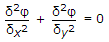# Civil Engineering - Hydraulics

### Exercise :: Hydraulics - Section 6

6.

Orifice-meter is used to measure

 A. pressure at the point B. discharge C. average speed D. velocity.

Explanation:

No answer description available for this question. Let us discuss.

7.

Pick up the correct statement from the following :

 A. Dimensional homogeneity means the dimensions of each term in an equation on both sides are equal B. Dimensionally homogeneous equations are independent of the system of units C. In dimensionally homogeneous equation, the powers of fundamental dimensions on either side of the equation are identical D. All the above.

Explanation:

No answer description available for this question. Let us discuss.

8.

The ratio of inertia force of a flowing fluid and the viscous force of the liquid is called :

 A. Renold's number B. Froude's number C. Euler's number D. Weber's number.

Explanation:

No answer description available for this question. Let us discuss.

9.

For an irrotational flow, the equationis given by

 A. Cauchy-Riemann B. Reynold C. Laplaces D. Bernoulli.

Explanation:

No answer description available for this question. Let us discuss.

10.

Water belongs to

 A. Newtonian fluids B. non-Newtonian fluids C. compressible fluid D. none of these.